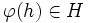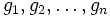# Verbal implies fully invariant

This article gives the statement and possibly, proof, of an implication relation between two subgroup properties. That is, it states that every subgroup satisfying the first subgroup property (i.e., verbal subgroup) must also satisfy the second subgroup property (i.e., fully invariant subgroup)
View all subgroup property implications | View all subgroup property non-implications
Get more facts about verbal subgroup|Get more facts about fully invariant subgroup

## Statement

If$H$ is a verbal subgroup of$G$, then$H$ is a fully invariant subgroup (also called a fully characteristic subgroup) in$G$.

## Definitions used

Term Definition used
verbal subgroup A subgroup$H$ of$G$ is a verbal subgroup if there exists a collection$C$ of words such that$H$ is the union of the images of the word maps corresponding to all the words in$C$.
fully invariant subgroup A subgroup$H$ of$G$ is a fully invariant subgroup if$\varphi(H) \le H$ for all endomorphisms$\varphi$ of$G$.

## Proof

### Hands-on proof

Given: A group$G$ and subgroup$H$.$C$ is a collection of words such that an element is in$H$ if and only if it can be expressed, as the value of a word in$C$ with letters taken as elements of$G$. In other words,$H$ is the union of the images of the word maps corresponding to the elements of$C$. (Note that the existence of such a$C$ is what makes$H$ a verbal subgroup). An endomorphism$\varphi$ of$G$ and element$h \in H$.

To prove:$\varphi(h) \in H$.

Step no. Assertion/construction Facts used Given data used Previous steps used Explanation
1 There exists$w \in C$ and elements$g_1,g_2, \ldots, g_n$ (where$n$ is the number of letters in$w$) such that$h = w(g_1,g_2,\ldots,g_n)$. From given condition on$H$ ($H$ being verbal) and$h \in H$.
2$\varphi(h) = \varphi(w(g_1,g_2,\ldots,g_n)) = w(\varphi(g_1),\varphi(g_2),\dots,\varphi(g_n))$ Fact (1)$\varphi$ is an endomorphism of$G$. Step (1) The second equality is direct from Fact (1), noting that an endomorphism of$G$ just means a homomorphism from$G$ to itself.
3$\varphi(h)$ is in the image of the word map corresponding to$w$, hence$\varphi(h) \in H$. Given condition on$H$. Steps (1), (2) Step (2) shows that$\varphi(h)$ is in the image of the word map corresponding to$w$, with inputs$\varphi(g_1), \dots \varphi(g_n)$. Step (1) reminds us that$w \in C$, so$\varphi(h) \in H$ by the definition of$H$.

### Slick proof

Note that any endomorphism is a homomorphism, so every endomomorphism of$G$ commutes with every word map of$G$ by Fact (1). Fact (2) now tells us that the image of every word map is invariant under every endomorphism of$G$. Since a union of invariant subsets under a bunch of functions is still invariant, we obtain that any union of images of word maps is invariant under every endomorphism. In particular, any verbal subgroup is invariant under every endomorphism.